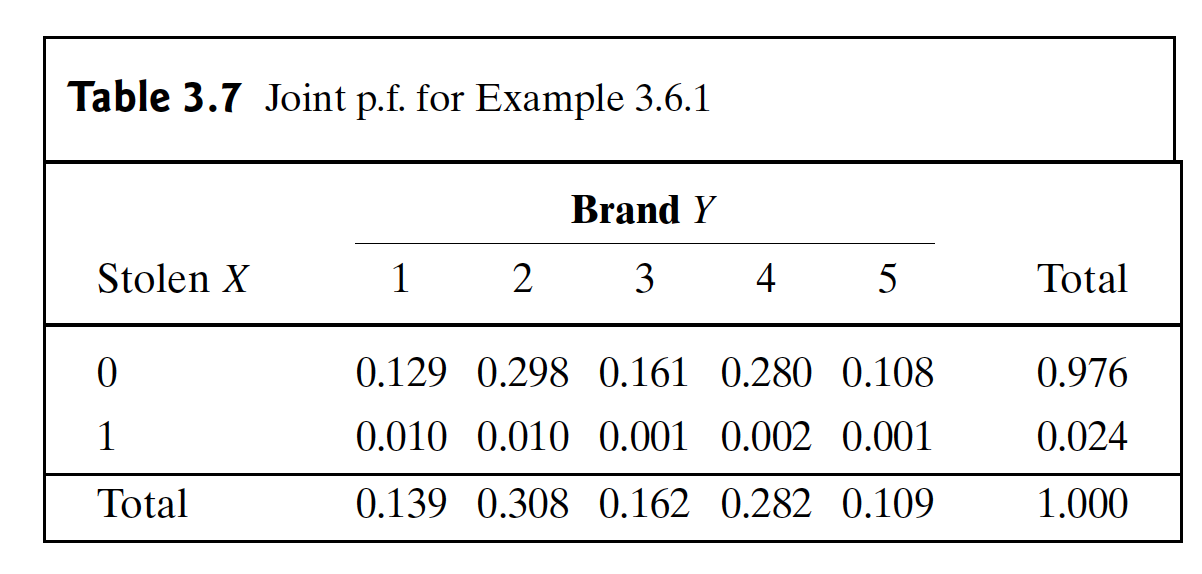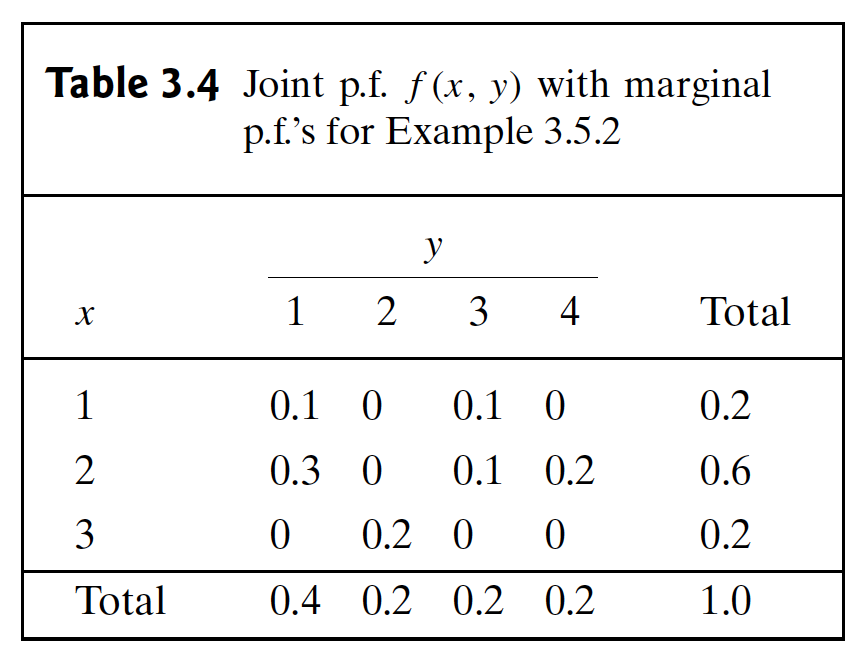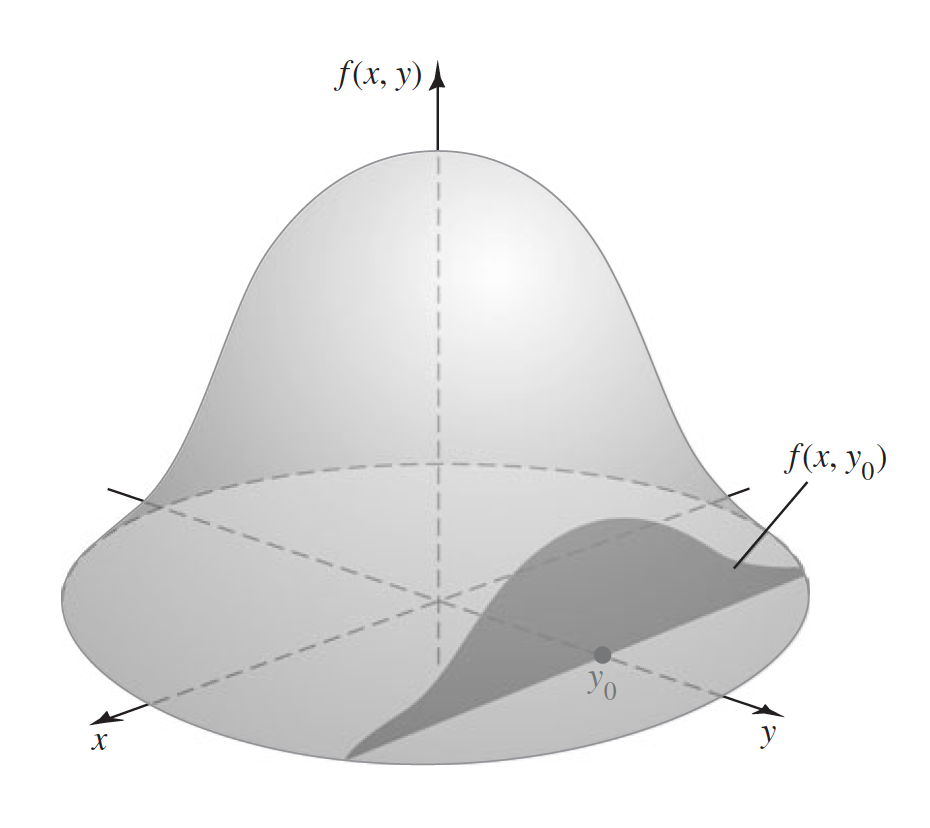Abstract: 首先介绍随机变量的条件分布，随后介绍随机变量条件分布下的乘法法则，贝叶斯公式和全概率公式
Keywords: Discrete Conditional Distributions，Continuous Conditional Distributions，Multiplication Rule，Bayes’ Therom，Law of Total Probability

# 条件分布

“如何变成一个真正的行家”

• 花几年时间进行紧张的学习，直到你觉得自己在行
• 离开几年，去探索更多其他的领域，无论是否有关
• 回到原来的领域，换个角度，重新掌握它
也许这与传统观点相悖，但你可以不用练习就升级，有时候这是升级的唯一办法

## 离散条件分布 Discrete Conditional Distributions$$Pr(X=x|Y=y)=\frac{Pr(X=x \text{ and } Y=y)}{Pr(Y=y)}=\frac{f(x,y)}{f_2(y)}$$

Definition Conditional Distribution/p.f. Let X and Y have a discrete joint distribution eith joint p.f. $f$ .Let $f_2$ denote the marginal p.f. of Y Fot each y such that $f_2(y)>0$ ,define:
$$g_1(x|y)=\frac{f(x,y)}{f_2(y)}$$
Then $g_1$ is called the conditional p.f. of X given Y.The discrete distribution whose p.f. is $g_1(\cdot |y)$ is called the conditional distribution of $X$ given that $Y=y$

$$\sum_x g_1(x|y)=\frac{1}{f_2(y)}\sum_xf(x,y)=\frac{1}{f_2(y)}f_2(y)=1$$$$g_2(y|2)=\frac{f(2,y)}{f_1(x=2)}=\frac{f(2,y)}{0.6}$$

## 连续条件分布 Continuous Conditional Distributions

$$f(x,y)= \begin{cases} e^{-x}&\text{ for }0\leq y\leq x<\infty &\\ 0 &\text{otherwise}& \end{cases}$$

Definition 3.6.2 p.d.f. :Let $X$ and $Y$ have a continuous joint distribution with joint p.d.f. $f$ and respective marginals $f_1$ and $f_2$ .Let $y$ be a value such that $f_2(y)>0$ .Then the conditional p.d.f. $g_1$ of $X$ given that $Y=y$ is defined as follows:
$$g_1(x|y)=\frac{f(x,y)}{f_2(y)}\text{ for }-\infty<x<\infty$$
For values of y such that $f_2(y)=0$ ,we are free to define $g_1(x|y)$ however we wish ,so long as $g_1(x|y)$ is a p.d.f. as a function of $x$

Theorem: For each $y$ , $g_1(x|y)$ defined in Definition 3.6.2 is a p.d.f. as a function of $x$

1. $f_2(y)=0$ 分母是0，没有计算意义
2. $f_2(y)>0$ 明显有$g_1(x|y)\geq0$
3. if $f_2(y)>0$
$$\int^{\infty}_{-\infty}g_1(x|y)dx=\frac{\int^{\infty}_{-\infty}f(x,y)dx}{f_2(y)}=\frac{f_2(y)}{f_2(y)}=1$$
Q.E.D

$$f_2(y)=\int^\infty_{y}e^{-x}dx=e^{-y}$$

$$g_1(x|y)=\frac{f(x,y)}{f_2(y)}=\frac{e^{-x}}{e^{-y}}=e^{y-x},\text{ for }x\geq y$$$$g_1(x|y)=lim_{\epsilon \to 0}\frac{\partial}{\partial x}Pr(X\leq x|y-\epsilon < Y \leq y+ \epsilon)$$

Definition Conditional p.f. or p.d.f. from Mixed Distribution: Let $X$ discrete and let $Y$ be continuous with joint p.f./p.d.f. f.Then the conditional p.f. of $X$ given $Y=y$ is defined by Eq.(3.6.2) and the conditional p.d.f of $Y$ given $X=x$ is defined by Eq.(3.6.3)

0%